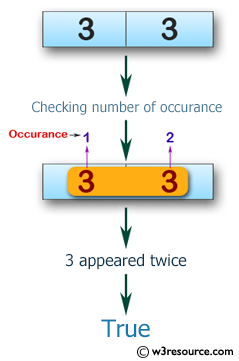﻿ Swift Array Programming Exercise: Check if a given array of integers contains 3 twice, or 5 twice - w3resource# Swift Array Programming Exercises: Check if a given array of integers contains 3 twice, or 5 twice

## Swift Array Programming: Exercise-14 with Solution

Write a Swift program to check if a given array of integers contains 3 twice, or 5 twice.

Pictorial Presentation:Sample Solution:

Swift Code:

``````func double_35(_ a: [Int]) -> Bool {
if a == [3, 3] || a == [5, 5]
{
return true
} else {
return false
}
}
print(double_35([3, 3]))
print(double_35([5, 5]))
print(double_35([1, 2]))
```
```

Sample Output:

```true
true
false
```

Swift Programming Code Editor:

Improve this sample solution and post your code through Disqus

What is the difficulty level of this exercise?

﻿